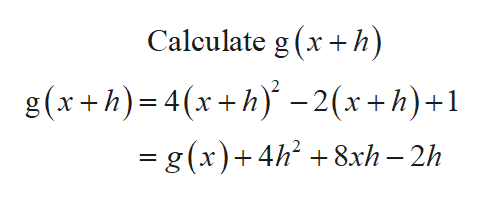Given g(x) = 4x2-2x+1, find (g(x+h) - g(x))/h.

Question

Given g(x) = 4x2-2x+1, find (g(x+h) - g(x))/h.

Step 1

Consider the given function:

Step 2help_outlineImage TranscriptioncloseCalculate g (xh) g(x+h) 4x+h-2(x+h)+1 = g (x)4h8xh - 2h fullscreen

Want to see the full answer?

See Solution

Want to see this answer and more?

Our solutions are written by experts, many with advanced degrees, and available 24/7

See Solution
Tagged in

Algebra